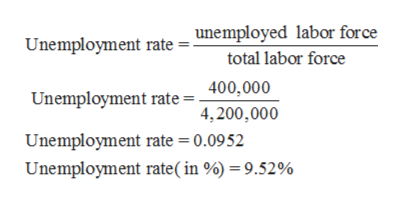Suppose that the adult population is 6 million, the number of employed is 3.8 million, and the labor-force participation rate is 70%. What is the unemployment rate?

Question
1. Suppose that the adult population is 6 million, the number of employed is 3.8 million, and the labor-force participation rate is 70%. What is the unemployment rate?
Step 1

Unemployment rate refers to the fraction or percentage of unemployed workforce from the total labor force.

Step 2

The total population is given to be 6,000,000.

Labor force participation rate is 70% or 0.70.

Hence, labor force participation can be calculated as:

Step 3

Now, number of employed labor force is 3,800,000

Hence, number of unemployed labor force is 4,2...help_outlineImage TranscriptioncloseUnemployment rate = unemployed labor force total labor force 400,000 Unemployment rate 4,200,000 Unemployment rate = 0.0952 Unemployment rate ( in %) = 9.52% fullscreen

Want to see the full answer?

See Solution

Want to see this answer and more?

Our solutions are written by experts, many with advanced degrees, and available 24/7

See Solution
Tagged in